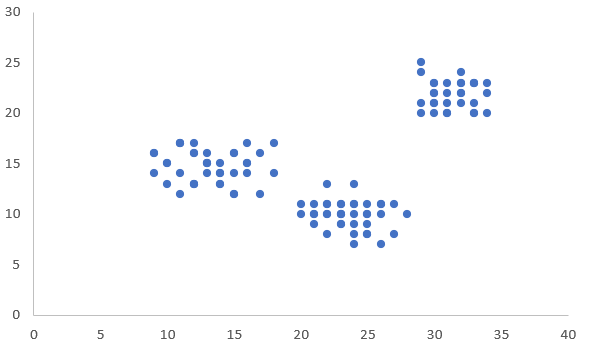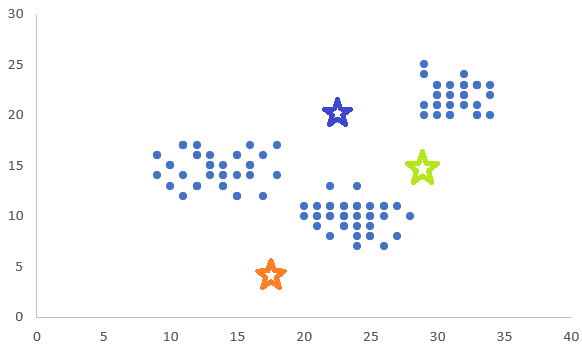top of page
Search
• Ekta Aggarwal

# K-Means Clustering

Updated: Aug 11, 2022

K-Means clustering is an unsupervised learning algorithm, which is used for clustering. This tutorial discusses in-depth explanation of K-Means algorithm.

To learn how to implement K-means algorithm in Python you can refer to this article: K Means Clustering in Python

### What is clustering?

Clustering is the process of grouping / segmenting the data in various groups, where each group has certain characteristics / speciality.

Clustering is widely used in customer segmentation in retail analytics. For eg., If you have the sales data for various customers, you can group the customers on the basis of the amount of the transactions, how frequently they are buying, and how recently they have made their last purchase.

Similarly in healthcare data, you can cluster / group the patients into various categories say, patients with high health risk (i.e., they are prone to getting more diseases in the future, say old patients with medical history), low health risk (i.e., they are not prone to getting fatal diseases in the future, say young and healthy people) etc.

To cluster the people we have several algorithms say, K-means clustering, K-medoids clustering, K-prototype, Hierarchical clustering, DB-Scan clustering, Gaussian Mixed Clustering etc.,

### K-Means Clustering Steps:

K-means clustering involves 2 major steps which we will discuss in detail:

1. Cluster allocation step

2. Move centroid step

Let us say we have 2 variables in our data and on plotting the graph looks as follows. Now we can clearly see that there are 3 groups of points in this data, but how does K-means algorithm works?

It is to be noted that for running K-means algorithm you need to define a value of 'K' i.e., number of clusters. For this example, let us say we want to build 3 clusters.Step 0: Since we want 3 clusters, thus we will choose any 3 random points (c1, c2 and c3) from our data and call them cluster centroids.

In this diagram, we have allocated 3 random points (illustrated as stars) as our cluster centroids.Cluster Allocation Step

Step a: Now we will take a point and calculate its Euclidean distance from all the 3 cluster centroids.

Now for a point, whichever centroid is closest to it, we will allocate it to that cluster.

Say, in the below image, for the point denoted by arrows, it has least Euclidean distance with 'orange' cluster centroid. Thus, it will belong to cluster say 'orange'.Similarly, suppose we have 1000 points in our data, then for each of the 1000 points we will calculate its Euclidean distance from the 3 cluster centroids. Whichever is the cluster centroid with least Euclidean Distance, we will allocate it to that cluster.

After cluster allocation, our data will look as follows after 1st iteration of clustering.Move Centroid Step

Since we had arbitrarily selected our cluster centroids, thus now on the basis of cluster allocation, we will 'move' or recalculate our cluster centroid.

The coordinates of new cluster centroid would be given by: Average of coordinates of points in the cluster.

Example, Suppose points with coordinates (a1,a2) and (b1,b2) belong to cluster 1. Thus co-ordinates of new cluster centroid would be: {(a1 + b1) / 2 , (a2 + b2) / 2 }

Thus, by taking the average co-ordinates of the points we will get our new cluster centroid.Step 3: Repeat Steps 1 and 2 until convergence is attained.

Since we have got new cluster centroids thus, we will re-allocate them like we did in 'cluster allocation step' and then again 'move the centroid' on the basis of the points in the clusters. We will keep on repeating it until the points in the clusters are no longer changing.

Thus, an optimal 'clustering' for this data would look as follows:### How to decide the number of clusters -

Number of clusters in K-means can be determined by business sense. For eg., let us say the business wants to have 3 or 4 clusters then we can use 3 or 4 clusters to implement K-means. Generally ideal number of clusters can be between 2 to 8.

2. Elbow method - widely used:

Earlier we had mentioned that for building a K-means clustering model, you would need to define the number of clusters. Thus, how do we decide about the number of clusters for our data?

For this we plot our cost function against the number of clusters,

The curve below looks like 'a human arm'. We can see that as the number of clusters are increasing our cost function is decreasing. But if we notice then our 'rate of decline' in the cost function is reducing tremendously when number of clusters = 4. Thus, it is forming an 'elbow' shaped curve, for number of clusters = 4.How do we calculate this cost function?

Let us say I have 3 clusters with centroids C1, C2, and C3. and I have 15 observations divided as:

1. Cluster 1: 5 observations

2. Cluster 2: 5 observations

3. Cluster 3: 5 observations

Now we calculate the square of Euclidean distance from its 'cluster centroid' and take an average of all these 15 distances. This will be our cost function or 'distortion'.

In the below table, X1 and X2 are 2 variables.

Column E represents the cluster number for each of these 15 points.

Columns F and G represent the co-ordinates of the cluster centroid.

Column H represents the squared Euclidean Distance. Calculation for cell H4 is elaborated in 'red text'

After calculating column H, we take average of these 15 distances, and thus, get our 'cost function' or 'distortion' as 9.47.### Standardising the variables

Since Euclidean distance can be influenced by difference in the scales of the variables (say one is in thousands, and other variable always taking the values less than 100), thus the variables are standardised to have mean 0 and variance 1 before K Means algorithm is implemented.

### Important Note!

Results of K-means depend on the initial cluster centroids. Thus, it is to be noted that once you have got the clusters, try to understand if you are able to differentiate the clusters. i.e., we try to draw the inferences of the variables after the clusters are formed.

For eg., In retail example, Cluster 1 contains customers with high transaction amount, while cluster 2 contains customers with lower transaction amount.

It is extremely essential to make business sense out of the clusters formed. Thus, once you have decided on the number of clusters, you can try experimenting the 'random seed' in the algorithm, which is used to determine the initial cluster centroids.

### Drawbacks:

1. You cannot use categorical variables: K - Means clustering does not incorporate categorical variables. One might argue that you can convert categorical variables into dummy variables as 0-1 notation, but that is also not justifiable. For eg., For the variable gender, say Male as 0 and Female as 1, but then it would adversely impact the calculation of Euclidean distances, as well as it won't be able to find the co-ordinate of new cluster centroid.

2. Highly influenced by outliers: Suppose an outlier is chosen as a cluster centroid at random, then the results of clustering would be highly unreliable. Similarly, existence of outliers can highly impact the calculation of Euclidean distances, thus impacting our calculation of new centroids after the clusters are allocated.

3. K-means performs poorly on the data with varying densities: K-means assumes that the clusters formed are spherical in nature and the densities are more or less similar. However, if the clusters are non-spherical in shape i.e., have varying densities then K-means would lead to bad results.

To learn how to implement K-means algorithm in Python you can refer to this article: K Means Clustering in Python

See All
bottom of page